## ML Aggarwal Class 6 Solutions for ICSE Maths Chapter 13 Practical Geometry Objective Type Questions

Mental Maths
Question 1.
Fill in the blanks:
(i) A ruler is used to draw line and to measure their …………
(ii) A divider is sued to compare …………
(iii) A compass is used to draw circles or arcs of …………
(iv) A protractor is used to draw and measure …………
(v) The set squares are two triangular pieces having angles of ………… and …………
(vi) To bisect a line segment of length 7 cm, the opening of the’ compass should be more than …………
(vii) The perpendicular bisector of a line segment is also its line of …………
Solution:
(i) A ruler is used to draw line segments and to measure their lengths.
(ii) A divider is sued to compare lengths of line segment.
(iii) A compass is used to draw circles or arcs of circles.
(iv) A protractor is used to draw and measure angles.
(v) The set squares are two triangular pieces having angles of
30°, 60°, 90° and 45°, 45°, 90°.
(vi) To bisect a line segment of length 7 cm,
the opening of the compass should be more than 3.5 cm.
(vii) The perpendicular bisector of a line segment is also its line of symmetry.

Question 2.
State whether the following statements are true (T) or false (F):
(i) There is only one set square in a geometry box.
(ii) An angle can be copied with the help of a ruler and compass.
(iii) The perpendicular bisector of a line segment can be drawn by paper folding.
(iv) Perpendicular to a line from a given point not on it can be drawn by paper folding.
(v) A 45° – 45° – 90° set square and a protractor have the same number of line(s) of symmetry.
Solution:
(i) There is only one set square in a geometry box. False
(ii) An angle can be copied with the help of a ruler and compass. True
(iii) The perpendicular bisector of a line segment
can be drawn by paper folding. True
(iv) Perpendicular to a line from a given point not on it
can be drawn by paper folding. True
(v) A 45° – 45° – 90° set square and a protractor have
the same number of line(s) of symmetry. True

Multiple Choice Questions
Choose the correct answer from the given four options (3 to 13):
Question 3.
A circle of any radius can be constructed with the help of a:
(a) ruler
(b) divider
(c) compass
(d) protractor
Solution:
compass (c)

Question 4.
The instrument in a geometry box having the shape of a semicircle is :
(a) ruler
(b) divider
(c) compass
(d) protractor
Solution:
protractor (d)
It is used to draw or measure angles.

Question 5.
The instrument to measure an angle is
(a) ruler
(b) protractor.
(c) divider
(d) compass
Solution:
protractor (b)

Question 6.
Which of the following angles cannot be constructed using ruler and compas?
(a) 15°
(b) 45°
(c) 75°
(d) 85°
Solution:
85° (d)

Question 7.
The number of perpendiculars that can be drawn to a line from a point not on it is
(a) 1
(b) 2
(c) 4
(d) infinitely many
Solution:
1 (a)

Question 8.
The number of perpendicular bisectors that can be drawn of a given line segment is :
(a) 0
(b) 1
(c) 2
(d) infinitely many
Solution:
1 (b)

Question 9.
The number of lines of symmetry in a picture of a divider is:The number of lines of symmetry in a picture of compass is
(a) 0
(b) 1
(c) 2
(d) 4
Solution:
1 (b)

Question 10.
The number of lines of symmetry in a picture of compass is
(a) 0
(b) 1
(c) 2
(d) none of these
Solution:
0 (a)

Question 11.
The number of lines of symmetry in a ruler is
(a) 0
(b) 1
(c) 2
(d) 4
Solution:
2 (c)

Question 12.
The number of lines of symmetry in a 30° – 60° – 90° set square is
(a) 0
(b) 1
(c) 2
(d) 3
Solution:
0 (a)

Question 13.
The number of lines of symmetry in a protractor is
(a) 0
(b) 1
(c) 2
(d) more than 2
Solution:
1 (b)

## ML Aggarwal Class 6 Solutions for ICSE Maths Chapter 13 Practical Geometry Check Your Progress

Question 1.
Draw a line segment AB = 5.4 cm. Construct a perpendicular at A by using ruler and compass.
Solution:
Steps of construction:

1. Draw AB = 5.4 cm.
2. With any radius draw an arc which cuts AB at M.
3. With M as centre and the same radius
cut the previous arc at N and P.
4. With N and P as centres draw arcs which intersect at L.
Join AL.
5. AL is required perpendicular.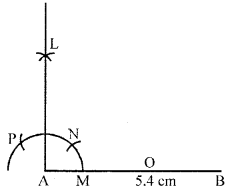Question 2.
Draw a line segment PQ = 6.8 cm. Draw a perpendicular to it from a point A outside PQ by using ruler and compass.
Solution: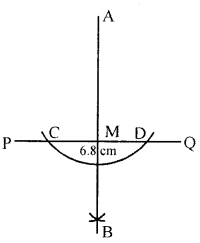Steps of construction:

1. Draw a line segment PQ = 6.8 cm
and take a point A outside PQ.
2. With A as centre and any suitable radius,
draw an arc to cut line PQ at point C and D.
3. With C and D as centres,
draw two arcs of equal radius cutting each other
at B on the other side of line PQ.
4. Join AB to meet the line PQ at M.

Question 3.
Draw a line segment of length 6.5 cm and construct its axis of symmetry.
Solution:
Steps of construction:

1. Draw a line segment $$\overline{\mathrm{AB}}$$ of length 6.5 cm.
2. With A as centre, using a compass, draw a circle.
The radius of this circle should be more than half of the length of $$\overline{\mathrm{AB}}$$.
3. With the same radius and with B as centre,
draw another circle using a compass.
Let it cut the previous circle at C and D.
4. Join CD. Then, $$\overline{\mathrm{CD}}$$ is the axis of symmetry of $$\overline{\mathrm{AB}}$$.Question 4.
Draw ∠AOB = 76° with help of a protractor. Bisect this angle by using ruler and compass. Measure the two parts by your protractor and see how accurate you are.
Solution:
Steps of construction:

1. Draw a line segment OB.
2. Construct ∠AOB with the help of protector = 76°.
3. With the help of compass and O as centre
draw an arc meeting OB and OA at P and Q respectively.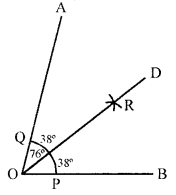4. With P and Q as centre and radius more than $$\frac{1}{2}$$ PQ
draw two arcs meeting each other at R.
5. OD is the bisector of ∠AOB.
6. On measuring ∠AOD = ∠DOB = 38°.

Question 5.
By using and compass, construct an angle of 135° and bisect it. Measure any one part by protractor and see how accurate you are.
Solution:
Steps of construction:

1. Draw a line OB with help of ruler.
2. With O as a centre and any suitable radius
draw an arc to meet OB at S.
3. With S as a centre and same radius
draw an arc to meet the previous arc at L.
With L as centre and same radius draw another arc M.
Again M as centre draws another arc to meet the first arc at N.
4. With M and N as centres draw two arcs of
equal radius $$\left(>\frac{1}{2} \mathrm{SL}\right)$$ cutting each other at A.
5. Join OA intersecting the radius at point Q.
6. Now taking Q and M as a centres
draw two arcs of equal radius cutting each other at P.
7. Join PO.
8. Measuring the ∠POB with protractor we get ∠POB equal to 135°.
9. Taking S and R as a centres draw two arcs cutting each other at T.
Join TO.
10. ∠TOB is the bisector of ∠POB. ∠TOB = ∠TOP = 67.5°.## ML Aggarwal Class 6 Solutions for ICSE Maths Chapter 13 Practical Geometry Ex 13.1

Question 1.
(i) 2 cm
(ii) 3.5 cm
Solution:
(a) 2 cm
Steps of construction :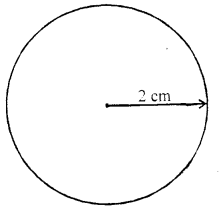(i) Open the compasses for the required radius 2 cm,
by putting the pointer on 0 and opening the pencil up to 2 cm
(ii) Draw a point with a sharp pencil and marks it as Q in the centre.
(iii) Place the pointer of the compasses where the centre has been marked.
(iv) Turn the compasses slowly to draw the circle.

(b) 3.5 cm
Steps of construction :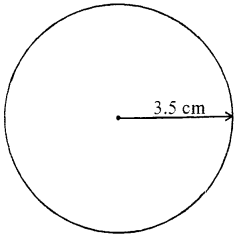(i) Open the compasses for the required radius 3.5cm
putting the pointer on 0 and openin’ g the pencil up to 3.5 cm
(ii) Draw a Point with a share Pencil and marks it as O in the centre.
(iii) Place the pointer of the compasses where the centre has been marked.
(iv) Turn the compasses slowly to draw the circle.

Question 2.
With the same centre O, draw two circles of radii 2.6 cm and 4.1 cm.
Solution:
Steps of construction :
(a) For a circle of radius 4.1 cm
(i) Pen the cor.npasses for the required radius 4.1 cm,
by putting the pointer on 0 and opening the pencil up to 4.1 cm.
(ii) Place the pointer of the compasses at 0.
(iii) Turn the compasses slowly t0 draw the circle.

(b) For a circle of radius of 2.6 cm
(i) Open the compasses for the required radius 2.6 cm,
by putting the pointer on 0 and opening the pencil up to 2.6 cm.
(ii) Place the pointer of the compasses at O.
(iii) Turn the compasses slowly to draw the circle.Question 3.
Draw any circle and mark points A, B and C such that
(i) A is on the circle.
(ii) B is in the interior of the circle.
(iii) C is in the exterior of the circle.
Solution: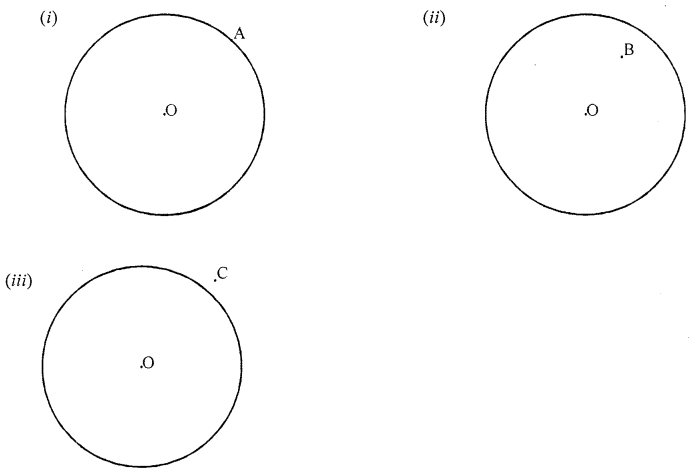Question 4.
Draw a circle and any two of its (non-perpendicular) diameters. If you join the ends of these diameters, what is the figure obtained? What figure is obtained if the diameters are perpendicular to each other? How do you check your answer?
Solution:
(i) On joining the ends of any two diameters of the circle,
the figure obtained is a rectangle.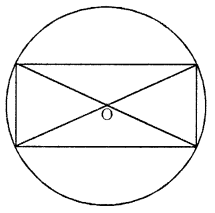(ii) On joining the ends of any two diameters of the circle,
perpendicular to each other, the figure obtained is a square.we measured the sides and angles of the figure obtained.

Question 5.
Let A, B be the centres of two circles of equal radii; draw them so that each one of them passes through the centre of the other. Let them intersect at C and D.
Examine whether $$\overline{\mathrm{AB}}$$ and $$\overline{\mathrm{CD}}$$ are at right angles.
Solution: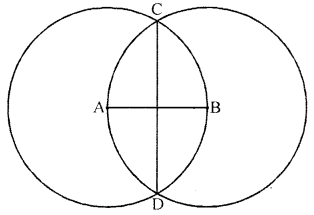Yes! $$\overline{\mathrm{AB}}$$ and $$\overline{\mathrm{CD}}$$ are at right angles.

Question 6.
Construct a line segment of length of 6.3 cm using ruler and compass.
Solution:
Using ruler, we mark two points A and B which are 7.3 cm apart.
Join A and B and get AB.
$$\overline{\mathrm{AB}}$$ is a line segment of length 7.3 cmQuestion 7.
Construct $$\overline{\mathrm{AB}}$$ of length 8.3 cm. From this cut off $$\overline{\mathrm{AC}}$$ of length 5.6 cm. Measure the length of BC . .
Solution:
Steps of construction :
(i) Draw a line l. Mark a point A on line l.
(ii) Place the compass pointer on the zero mark of the ruler.
Open it to place the pencil point upto the 8.3 cm mark.
(iii) Without changing the opening of the compass,
place the pointer on A and swing an arc to cut l at B.
(iv) $$\overline{\mathrm{AB}}$$ is a line segment of required of length 8.3 cm.
(v) Place the compass pointer on the zero mark of the ruler.
Open it to place the pencil point upto 5.6 cm mark.
(vi) Withtout changing the opening of the compass,
place the pointer on A and swing ana rc to cut l at C.
(vii) $$\overline{\mathrm{AC}}$$ is a line segment of length 5.6 cm.
On measurement, $$\overline{\mathrm{BC}}$$ = 2.7 cm.Question 8.
Draw any line segment $$\overline{\mathrm{PQ}}$$. Without measure $$\overline{\mathrm{PQ}}$$, construct a copy of $$\overline{\mathrm{PQ}}$$.
Solution:
(i) Given $$\overline{\mathrm{PQ}}$$ whose length is not known.
(ii) Fix the compass pointer on P and the pencil end on Q.
The opening of the instrument now gives the length of $$\overline{\mathrm{PQ}}$$.
(iii) Draw any line l. Choose a point A on l.
Without changing the compass setting, place the pointer on A.
(iv) Swing an arc that cuts l at a point, say, B. Now AB is a copy of $$\overline{\mathrm{PQ}}$$.

Question 9.
Given some line segment $$\overline{\mathrm{AB}}$$, whose length you do not know, construct $$\overline{\mathrm{PQ}}$$ such that the length of $$\overline{\mathrm{PQ}}$$ is twice that of $$\overline{\mathrm{AB}}$$.
Solution:
(i) Given $$\overline{\mathrm{AB}}$$ whose length is not known.
(ii) Fix the compass pointer on A and the pencil end on B.
The opening of the instrument now gives the length of AB.
(iii) Draw any line 1. Choose a point P on l.
Without changing the compass setting, place the pointer on P.
(iv) Strike an arc that cuts l at a point, say, X.
(v) Now fix the compass pointer on X.
Strike an arc away from P that cuts l at a point, say, Q.
Now, the length of $$\overline{\mathrm{PQ}}$$ is twice that of AB.

Question 10.
Take a line segment $$\overline{\mathrm{PQ}}$$ of length 10 cm. From $$\overline{\mathrm{PQ}}$$, cut of $$\overline{\mathrm{PA}}$$ of length 4.3 cm and $$\overline{\mathrm{BQ}}$$ of length 2.5 cm. Measure the length of segment $$\overline{\mathrm{AB}}$$.
Solution: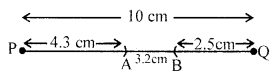∴ Length of $$\overline{\mathrm{AB}}$$ is 3.2 cm.

Question 11.
Given two line segments $$\overline{\mathrm{AB}}$$ and $$\overline{\mathrm{CD}}$$ of length 7.5 cm and 4.6 respectively. Construct line segments.
(i) $$\overrightarrow { PQ }$$ of length equal to the sum of the lengths of $$\overline{\mathrm{AB}}$$ and $$\overline{\mathrm{CD}}$$.
(ii) $$\overline{\mathrm{XY}}$$ of length equal to the difference of the lengths of $$\overline{\mathrm{AB}}$$ and $$\overline{\mathrm{CD}}$$. Verify these lengths by measurements.
Solution:(ii) $$\overline{\mathrm{XY}}$$ = $$\overline{\mathrm{AB}}$$ – $$\overline{\mathrm{CD}}$$ = 7.5 cm – 4.6 cm = 2.9 cm

## ML Aggarwal Class 6 Solutions for ICSE Maths Chapter 13 Practical Geometry Ex 13.2

Question 1.
Draw a line segment $$\overline{\mathrm{PQ}}$$ =5.6 cm. Draw a perpendicular to it from a point A outside $$\overline{\mathrm{PQ}}$$ by using ruler and compass.
Solution:
Given: A-Line segment PQ = 5.6 cm and a point A outside the line.
Required: To draw a 1 ar to PQ from point A.
Steps of construction :
(i) With A as centre and any suitable radius,
drawn an arc to cut the line PQ at points C and D.
(ii) With C and D as centres, drawn two arcs of equal radius $$\left(>\frac{1}{2} \mathrm{CD}\right)$$
cutting each other at B on the other side of PQ.
(iii) Join A and B to meet the line PQ at N,
then AN is the required perpendicular from the point A to the line PQ.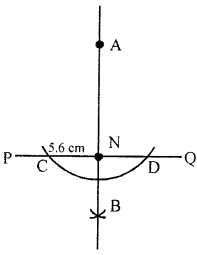Question 2.
Draw a line segment $$\overline{\mathrm{AB}}$$ = 6.2 cm. Draw a perpendicular to it at a point M on $$\overline{\mathrm{AB}}$$ by using ruler and compass.
Solution:
Given: A line AB = 6.2 cm and a point P on it.
Required: To draw an ⊥ arc to AB at point P.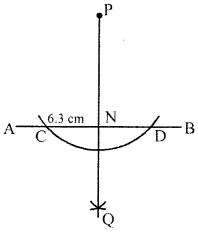Step of Construction :
(i) With P as centre and any suitable radius,
draw an arc to cut the line AB at points C and D.
(ii) With C and D as centres,
draw two arcs of equal radius $$\left(>\frac{1}{2} \mathrm{CD}\right)$$ cutting each other at Q.
(iii) Join P and Q.
then QP is the required perpendicular to the line AB at the point P.

Question 3.
Draw a line l and take a point P on it. Through P, draw a line segment $$\overline{\mathrm{PQ}}$$ perpendicular to l. Now draw a perpendicular to $$\overline{\mathrm{PQ}}$$ at Q (use ruler and compass).
Solution:
Steps of construction :
(i) Let AB be the given line segment.
(ii) With A as centre and any suitable radius $$\left(>\frac{1}{2} \mathrm{CD}\right)$$
draw arcs on each side of AB.
(iii) With B as centre and same radius [as in step (i)],
draw arcs on each side of AB to cut the previous arcs at P and Q.
(iv) Draw a line passing through points P and Q,
then the lines $$\overline{\mathrm{PQ}}$$ is the required perpendicular bisector of AB and line l.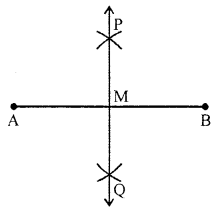Question 4.
Draw a line segment $$\overline{\mathrm{AB}}$$ of length 6.4 cm and construct its axis of symmetry (use ruler and compass).
Solution:
Steps of construction :
(i) Draw a line segment $$\overline{\mathrm{AB}}$$ of length 6.4 cm.
(ii) With A as centre, using a compass, draw a circle.
The radius of this circle should be more than half of the length of AB.
(iii) With the same radius and with B as centre,
draw another circle using a compass.
Let it cut the previous circle at C and D.
(iv) Join $$\overline{\mathrm{CD}}$$. Then, $$\overline{\mathrm{CD}}$$ is the axis of symmetry of $$\overline{\mathrm{AB}}$$.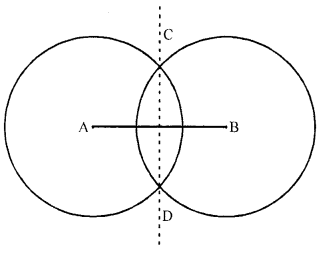Question 5.
Draw the perpendicular bisector of $$\overline{\mathrm{XY}}$$ whose length is 8.3 cm.
(i) Take any point P on the bisector drawn. Examine whether PX = PY.
(ii) If M is the mid-point of $$\overline{\mathrm{XY}}$$, what can you say about the lengths MX and MY?
Solution:
Steps of construction :
(i) Draw a line segment $$\overline{\mathrm{XY}}$$ of length 8.3 cm.
(ii) With X as centre, using compass, draw a circle.
The radius of this circle should be more than half of the length of $$\overline{\mathrm{XY}}$$.
(iii) With the same radius and with Y as centre,
draw another circle using a compass.
Let it cut the previous circle at A and B.
(iv) Join AB.
Then, $$\overline{\mathrm{AB}}$$ is the perpendicular bisector of the line segment $$\overline{\mathrm{XY}}$$.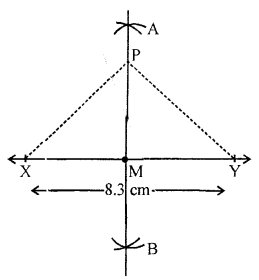(a) On examination, we find the PX = PY.
(b) We can say that the length of MX is Equal to the length of MY.

Question 6.
Draw a line segment of length 8.8 cm. Using ruler and compass, divide it into four equal parts. Verify by actual measurement.
Solution:
Steps of construction :
(i) Draw a line segment $$\overline{\mathrm{AB}}$$ of length 8.8 cm.
(ii) With A as centre, using compass,
draw two arcs on either side of AB.
The radius of this arc should be more than half of the length of $$\overline{\mathrm{AB}}$$.
(iii) With the same radius and with B as ctntre,
draw another arc using compass.
Let it cut the previous arc at C and D.
(iv) Join $$\overline{\mathrm{CD}}$$.
It cuts $$\overline{\mathrm{AB}}$$ at E.
Then $$\overline{\mathrm{CD}}$$ is the perpendicular bisector of the line segment $$\overline{\mathrm{AB}}$$.
(v) With A as centre, using compass, draw a circle.
The radius of this circle stould be more than half of the length of Ac.
(vi) With the same radius and with E as ceitre,
draw another circle using compass.
Let it cut the previous circle at F ana G.
(vii) Join $$\overline{\mathrm{FG}}$$ . It cuts $$\overline{\mathrm{AE}}$$ at H.
Then $$\overline{\mathrm{FG}}$$ is the perpendicular bisector of the line segment $$\overline{\mathrm{AE}}$$.
(viii) With E as centre, using eompass, draw a circle.
The radius of thii circle slould be more than half of the length of EB.
(ix) With the same radius md with B is centre,
draw another circle using compss.
Let it cut the previous cirde at I and J.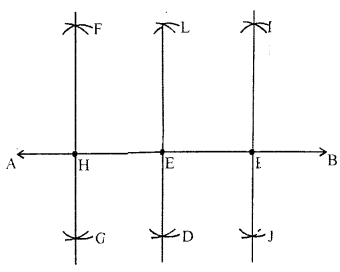(x) Join $$\overline{\mathrm{IJ}}$$ . It cuts $$\overline{\mathrm{EB}}$$ at K.
Then $$\overline{\mathrm{IJ}}$$ is the perpendicuir bisector of the lhe segment $$\overline{\mathrm{EB}}$$.
Now, the points H, E and K divide AB into four equal parts. i. e.,
$$\overline{\mathrm{AH}}$$ = $$\overline{\mathrm{HE}}$$ = $$\overline{\mathrm{EK}}$$ = $$\overline{\mathrm{KB}}$$
By measurement,
$$\overline{\mathrm{AH}}$$ = $$\overline{\mathrm{HE}}$$ = $$\overline{\mathrm{EK}}$$ = $$\overline{\mathrm{KB}}$$ = 2.2 cm

Question 7.
With $$\overline{\mathrm{PQ}}$$ of length 5.6 cm as diameter, draw a circle.
Solution:
Steps of construction :
(i) Draw a line segment $$\overline{\mathrm{PQ}}$$ of length 5.6 cm.
(ii) With P as centre, using compass, draw a circle.
The radius of this circle should be more than half of the length of $$\overline{\mathrm{PQ}}$$.
(iii) With the same radius and with Q as centre,
draw another circle using compass.
Let it cut the previous circle at A and B.
(iv) Join $$\overline{\mathrm{AB}}$$. It cuts $$\overline{\mathrm{PQ}}$$ at C.
Then AB is the perpendicular bisector of the line segment $$\overline{\mathrm{PQ}}$$.
(v) Place the pointer of the compass at C
and open the pencil up to P.
(vi) Turn the compass slowly to draw the circle.Question 8.
Draw a circle with centre C and radius 4.2 cm. Draw any chord AB. Construct the perpendicular bisector of AB and examine if it passes through C.
Solution:
Steps of construction :
(i) Draw a point with a sharp pencil aid mark it as C.
(ii) Open the compass for the required radius of 4.2 cm,
by putting the pointer on 0 and opening the pencil up to 4.2 cm.
(iii) Place the pointer of the compass at C.
(iv) Turn the compass slowly to draw the circle.
(v) Draw any chord $$\overline{\mathrm{AB}}$$ of this circle.
(vi) With A as centre, using compass, draw a circle.
The radius of this circle should be more than half of the length of $$\overline{\mathrm{AB}}$$.
(vii) With the same radius and with B as centre,
draw another circle using compass.
Let it cut the previous circle at D and E.
(viii) Join $$\overline{\mathrm{DE}}$$.
Then $$\overline{\mathrm{DE}}$$ is the perpendicular bisector of the line segment $$\overline{\mathrm{AB}}$$.
On examination, we find that it passes through C.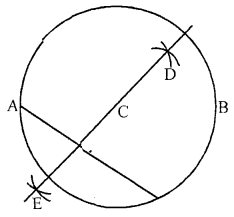Question 9.
Draw a circle of radius 3.5 cm. Draw any two of its (non-parallel) chords. Construct the perpendicular bisectors of these chords. Where do they meet?
Solution:
Steps of construction :
(i) Draw a point with a sharp pencil and mark it as O.
(ii) Open the compasses for the required radius 3.5 cm,
by putting the pointer on 0 and opening the pencil upto 3.5 cm.
(iii) Place the pointer of the compass at O.
(iv) Turn the compass slowly to draw the circle.
(v) Draw any two chords $$\overline{\mathrm{AB}}$$B and $$\overline{\mathrm{CD}}$$ of this circle.
(vi) With A as centre, using compass, draw two arcs on either side of AB.
The radius of this arc should be more than half of the length of $$\overline{\mathrm{AB}}$$.
(vii) With the same radius and with B as centre,
draw another two arcs using compass.
Let it cut the previous circle at E and F.
(viii) Join $$\overline{\mathrm{EF}}$$.
Then $$\overline{\mathrm{EF}}$$ is the perpendicular bisector of the chord $$\overline{\mathrm{AB}}$$.
(ix) With C as centre, using compass,
draw two arcs on either side of CD.
The radius of this arc should be more than half of the length of $$\overline{\mathrm{CD}}$$.
(x) With the same radius and with D as centre,
draw another two arcs using a compass.
Let it cut the previous circle at G and H.
(xi) Join $$\overline{\mathrm{GH}}$$.
Then $$\overline{\mathrm{GH}}$$ is the perpendicular bisector of the chord $$\overline{\mathrm{CD}}$$.
We find that perpendicular bisectots $$\overline{\mathrm{EF}}$$ and $$\overline{\mathrm{GH}}$$ meet at O,
the centre of the circle.Question 1.
Draw an angle of 80° and make a copy of it using ruler and compass.
Solution:
Steps of construction :
(i) Construct an angle ABC = 80°.
(ii) Take a line l and mark a point D on it.
(iii) Fix the compass pointer on B and
draw an arc which cuts the sides of ∠ABC at D and E.
(iv) Without changing the compass setting,
place the pointer on P and draw an arc which cuts l at Q.
(v) Open the compass equal to length DE.
(vi) Without disturbing the radius on compass,
place its pointer at Q and draw an arc which cuts the previous arc at R.
(vii) Join PR and draw ray PR.
Its gives ∠RPQ which is the required angle
whose measure is equal to the measure of ∠ABC.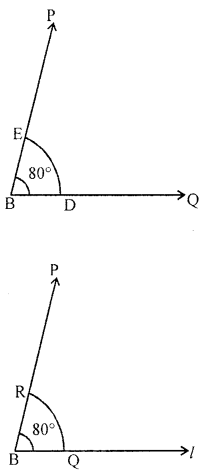Question 2.
Draw an angle of measure 127° and construct its bisector.
Solution:
Steps of construction :
(i) Draw $$\overline{\mathrm{OQ}}$$ of any length.
(ii) Place the centre of the protractor at O and the zero edge along $$\overline{\mathrm{OQ}}$$.
(iv) Join $$\overline{\mathrm{OP}}$$. Then, ∠POQ = 127°
(v) With O as centre and using compass,
draw an arc that cuts both rays of ∠POQ.
Label the points of intersection as P’ and Q’.
(vi) With Q’ as centre, draw (in the interior of ∠POQ)
an arc whose radius is more than half the length Q’P’.
(vii) With the same radius and with P’ as centre,
draw another arc in the interior of ∠POQ.
Let the two arcs intersect at R. Then, $$\overline{\mathrm{OR}}$$ is the bisector of ∠POQ.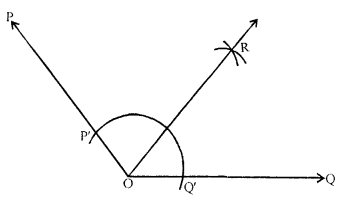Question 3.
Draw ∠POQ = 64°. Also draw its line of symmetry.
Solution:
Steps of construction :
(i) Draw a ray $$\overline{\mathrm{OQ}}$$
(ii) Place the centre of the protractor at O and the zero edge along $$\overline{\mathrm{OQ}}$$.
(iv) Join $$\overline{\mathrm{OP}}$$ . Then, ∠POQ = 64°.
(v) With O as centre and using compass,
draw an arc that cuts both rays of ∠POQ.
Label the points of intersection as P’ and Q’.
(vi) With Q’ as centre, draw (in the interior of ∠POQ)
an arc whose radius is more than half the length Q’P’.
(vii) With the same radius and with P’ as centre,
draw another arc in the interior of ∠POQ.
Let the two arcs intersect at R.
Then, $$\overline{\mathrm{OR}}$$ is the bisector of ∠POQ
which is also the line of symmetry of ∠POQ as ∠POR = ∠ROQ.Question 4.
Draw a right angle and construct its bisector.
Solution:
Steps of construction :
(i) Draw a ray OQ.
(ii) Place the centre of the protractor at O and the zero edge along $$\overline{\mathrm{OQ}}$$.
(iv) Join $$\overline{\mathrm{OP}}$$. Then, ∠POQ = 90°
(v) With 0 as centre and using compass,
draw an arc that cuts both rays of ∠POQ.
Label the points of intersection as P’ and Q’.
(vi) With Q’ as centre, draw (in the interior of ∠POQ)
an arc whose radius is more than half the length Q’P’.
(vii) With the same radius and with P’ as centre,
draw another arc in the interior of ∠POQ.
Let the two arcs intersect at R.
Then, $$\overline{\mathrm{OR}}$$ is the bisector of ∠POQ.Question 5.
Draw an angle of 152° and divide it into four equal parts.
Solution:
Steps of construction :
(i) Draw a ray $$\overline{\mathrm{OQ}}$$.
(ii) Place the centre of the protractor at O and the zero edge along $$\overline{\mathrm{OQ}}$$.
(iii) Start with 0 near Q. Mark a point P at 152°.
(iv) Join OP. Then, ∠POQ =152°
(v) With O as centre and using compass,
draw an arc that cuts both rays of ∠POQ.
Label the points of intersection as P’ and Q’.
(vi) With Q’ as centre, draw (in the interior of ∠POQ)
an arc whose radius is more than half the length Q’P’.
(vii) With the same radius and with P’ as centre,
draw another arc in the interior of ∠POQ.
Let the two arcs intersect at R. Then, $$\overline{\mathrm{OR}}$$ is the bisector of ∠POQ.
(viii)With O as centre and using compasses,
draw an arc that cuts both rays of ∠ROQ.
Label the points of intersection as B and A.
(ix) With A as centre, draw (in the interior of ∠ROQ)
an arc whose radius is more than half the length AB.
(x) With the same radius and with B as centre,
draw another arc in the interior of ∠ROQ.
Let the two arcs intersect at S. Then, $$\overline{\mathrm{OS}}$$ is the bisector of ∠ROQ.
(xi) With O as centre and using compass,
draw an arc that cuts both rays of ∠POR.
Label the points of intersection as D and C.
(xii) With C as centre, draw (in the interior of ∠POR)
an arc whose radius is more than half the length CD.
(xiii) With the same radius and with D as centre,
draw another arc in the interior of ∠POR.
Let the two arcs intersect at T.
Then, $$\overline{\mathrm{OT}}$$ is the bisector of ∠POR.
Thus, $$\overline{\mathrm{OS}}$$, $$\overline{\mathrm{OR}}$$ and $$\overline{\mathrm{OT}}$$ divide ∠POQ = 152° into four equal parts.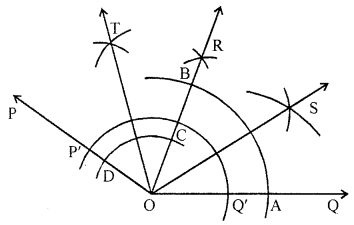Question 6.
Draw an angle of measure 45° and bisect it.
Solution:
Steps of construction :
(i) Draw a straight line BC.
(ii) With B as a centre and any suitable radius,
draw an arc to meet BC at E.
(iii) With E as centre and same radius
draw an arc to meet the previous arc at G.
(iv) With G and F as centre and same radius
draw another arc to meet the first arc at H.
(v) With H and E as centre draw two arcs of equal radius less than $$\frac{1}{2}$$ GE.
(vi) Cutting each other at J joined BJ and produce it to D.
(vii) With L and E as centre draw two arcs of equal radius less than $$\frac{1}{2}$$ LE.
(viii) Cutting each other at K joined BK and produce it to I.
(ix) Measuring angle ∠IBC = 22.5°